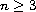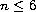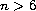Electron. J. Diff. Eqns., Vol. 2005(2005), No. 118, pp. 1-28.

### Stability of energy-critical nonlinear Schrodinger equations in high dimensions Terence Tao, Monica Visan

Abstract:
We develop the existence, uniqueness, continuity, stability, and scattering theory for energy-critical nonlinear Schrodinger equations in dimensions, for solutions which have large, but finite, energy and large, but finite, Strichartz norms. For dimensions, this theory is a standard extension of the small data well-posedness theory based on iteration in Strichartz spaces. However, in dimensionsthere is an obstruction to this approach because of the subquadratic nature of the nonlinearity (which makes the derivative of the nonlinearity non-Lipschitz). We resolve this by iterating in exotic Strichartz spaces instead. The theory developed here will be applied in a subsequent paper of the second author, , to establish global well-posedness and scattering for the defocusing energy-critical equation for large energy data.

Submitted July 2, 2005. Published October 26, 2005.
Math Subject Classifications: 35J10.
Key Words: Local well-posedness; uniform well-posedness; scattering theory; Strichartz estimates

Show me the PDF file (405K), TEX file, and other files for this article.Terence Tao Department of Mathematics University of California Los Angeles, CA 90095-1555, USA email: tao@math.ucla.edu Monica Visan Department of Mathematics University of California Los Angeles, CA 90095-1555, USA email: mvisan@math.ucla.edu